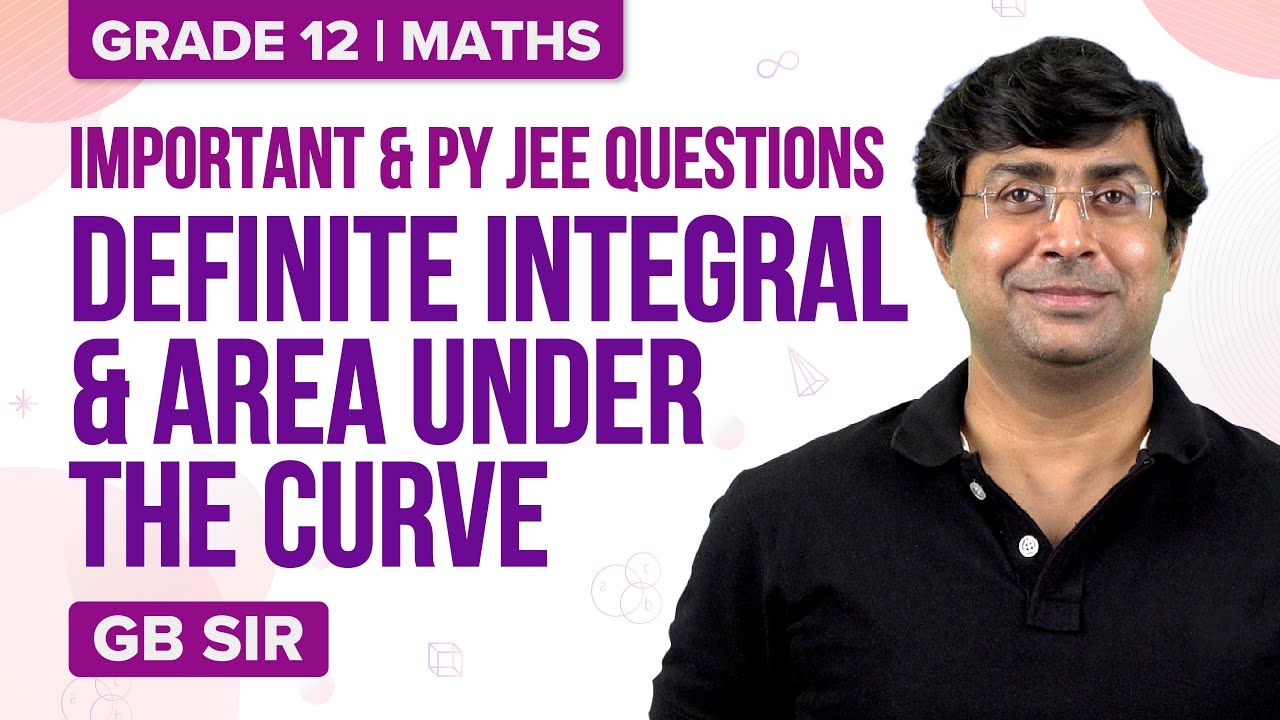Checkout JEE MAINS 2022 Question Paper Analysis : Checkout JEE MAINS 2022 Question Paper Analysis :

# JEE Advanced Maths Definite Integration Previous Year Questions with Solutions

Definite Integration JEE Advanced previous year questions with solutions are given on this page. These solutions are prepared by our subject experts. Evaluation of definite integrals by substitution, properties of definite integrals, reduction formulae for definite integration, Walli’s formula, summation of series by integration, etc are the important topics in definite integration.

Aspirants who are preparing for the JEE Advanced are recommended to go through these solutions, so that they can understand the difficulty level and question pattern.

Question 1: The value of the integral

$$\begin{array}{l}\int_{0}^{\frac{1}{2}}\frac{1+\sqrt{3}}{((x+1)^{2}(1-x)^{6})^{\frac{1}{4}}}dx\end{array}$$
is

(a) 2

(b) -2

(c) 1

(d) 3

Solution:

Let I = ∫01/2 [(1+√3)/(1+x)2(1-x)6]1/4 dx

= ∫01/2 (1+√3)/(1+x)2[(1-x)6/(1+x)6]1/4 dx

Put (1-x)/(1+x) = t

=> -2dx/(1+x)2 = dt

So I = ∫11/3(1+√3)/-2t6/4 dt

= [-(1+√3)/2]|-2/√t|11/3

= (√3+1)(√3-1)

= 2

Hence option a is the answer.

Question 2: The integral ∫12 ex xx (2+logex) dx equals

(a) e(4e+1)

(b) 4e2 – 1

(c) e(4e-1)

(d) e(2e-1)

Solution:

Let I = ∫12 ex xx (2+logex) dx

I = ∫12 ex xx [1 + (1+logex)] dx

= ∫12 ex [xx  + xx(1+logex)] dx

Since ∫ ex [f(x) +f’(x)] dx = ex f(x) + c

I = [ex xx]12

= e2×4 – e×1

= 4e2 – e

= e(4e – 1)

Hence option c is the answer.

Question 3: The value of

$$\begin{array}{l}\int_{\frac{-\pi }{2}}^{\frac{\pi }{2}}\frac{x^{2}\cos x}{1+e^{x}}dx\end{array}$$
is equal to

(a) π2/4 – 2

(b) π2/4 + 2

(c) π2/4

(d) 0

Solution:

Let I =

$$\begin{array}{l}\int_{\frac{-\pi }{2}}^{\frac{\pi }{2}}\frac{x^{2}\cos x}{1+e^{x}}dx\end{array}$$

=

$$\begin{array}{l}\int_{\frac{-\pi }{2}}^{\frac{\pi }{2}}\frac{x^{2}\cos x}{1+e^{-x}}dx\end{array}$$

I =

$$\begin{array}{l}\int_{\frac{-\pi }{2}}^{\frac{\pi }{2}}\frac{e^{x}x^{2}\cos x}{1+e^{x}}dx\end{array}$$

Since ∫abf(x) dx = ∫abf(a+b-x) dx

2I = ∫-π/2π/2 x2 cos x dx = 2∫0π/2 x2 cos x dx

I = [x2 sin x + 2x cos x – 2 sin x]0π/2

= π2/4 – 2

Hence option a is the answer.

Question 4: The value of ∫01 4x3 (d2/dx2)(1-x2)5 dx is

(a) 2

(b) 1

(c) 3

(d) 0

Solution:

Use integration by parts

01 4x3 (d2/dx2)(1-x2)5 dx = [4x3 (d/dx)(1-x2)5 ]01 – ∫0112x2 (d/dx)(1-x2)5 dx

= [4x3 ×5(1-x2)(-2x)]01 – 12 [[x2(1-x2)5]01 – ∫01 2x(1-x2)5 dx]

= 0 – 0 – 12 (0-0) + 12 ∫01 2x(1-x2)5 dx]

= 12×[-(1-x2)6/6]01

= 12(0+⅙)

= 2

Hence option a is the answer.

Question 5: The value of ∫1e (tan-1x/x + log x/(1+x2)) dx is

(a) tan e

(b) tan-1 e

(c) tan-1 1/e

(d) none of these

Solution:

Integrating by parts.

Take tan-1 x as first function and 1/x as second function.

1e (tan-1x/x + log x/(1+x2)) dx = [tan-1x log x]1e – ∫1elog x/(1+x2) dx + ∫1elog x/(1+x2) dx

= tan-1 e

Hence option b is the answer.

Question 6: The value of the integral ∫-π/2π/2 (x2 + ln (π+x)/(π-x)) cos x dx is

(a) 0

(b) π2/2 – 4

(c) π2/2 + 4

(d) π2/2

Solution:

-π/2π/2 [x2 + ln (π+x)/(π-x)] cos x dx = ∫-π/2π/2 x2 cos x dx + ∫-π/2π/2 ln ((π+x)/(π-x)) cos x dx

= 2∫0π/2 x2 cos x dx + 0 (since x2 cos x is even function and ln (π+x)/(π-x) cos x is an odd function.)

= 2[x2 sin x + 2x cos x – 2 sin x]0π/2

= π2/2 – 4

Hence option b is the answer.

Question 7: Let f:R→ R be a continuous function which f(x) = ∫0x f(t) dt. Then the value of f(ln 5) is

(a) 1

(b) 0

(c) 2

(d) 4

Solution:

f(x) = ∫0x f(t) dt

f’(x) = (d/dx)∫0x f(t) dt

Using Leibnitz theorem, we get

= (d/dx) f(x).x – (d/dx) f(0).0

= f(x)

f’(x)/f(x) = 1

Integrating both sides

ln f(x) = x + c

=> f(x) = ex+c

= ex. ec

Let ec = A

Then f(x) = Aex

f(0) = ∫00 f(t) dt = 0

When x = 0, y = 0, A = 0

=> f(x) = 0

f(ln 5) = 0

Hence option b is the answer.

Question 8: Let f: [0, 2] → R be a function which is continuous on [0, 2] and its differentiable on (0, 2) with f(0) = 1. Let F(x) =

$$\begin{array}{l}\int_{0}^{x^{2}}f(\sqrt{t})dt\end{array}$$
for x∈ [0, 2]. If F(x) = f’(x) for all x belongs to (0, 2), then F(2) equals

(a) e2 – 1

(b) e4 – 1

(c) e – 1

(d) e4

Solution:

F(x) =

$$\begin{array}{l}\int_{0}^{x^{2}}f(\sqrt{t})dt\end{array}$$

F’(x) = f(x) 2x

F’(x) = f’(x) for all x belongs to (0, 2)

=> f(x) 2x = f’(x)

=> f’(x)/f(x) = 2x

On integrating w.r.t.x,we get

ln f(x) = x2 + c

f(x) =

$$\begin{array}{l}e^{x^{2}+c}\end{array}$$

=

$$\begin{array}{l}e^{x^{2}}.e^{c}\end{array}$$

Since f(0) = 1

=> ec = 1

=> f(x) =

$$\begin{array}{l}e^{x^{2}}\end{array}$$

Hence F(x) =

$$\begin{array}{l}\int_{0}^{x^{2}}e^{x}dx\end{array}$$

=

$$\begin{array}{l}e^{x^{2}}-1\end{array}$$

F(2) = e4 – 1

Hence option b is the answer.

Question 9: The total number of distinct x ∈ [0, 1] for which ∫0x t2/(1+t4) dt = 2x-1 is

Solution:

Let f(x) = ∫0x t2/(1+t4) dt – 2x+1

f’(x) = x2/(1+x4) – 2 < 0 for all x ∈ [0, 1]

So f is decreasing on [0, 1]

Also f(0) = 1

f(1) = ∫01 t2/(1+t4) dt – 1

For 0≤ t ≤ 1

0≤ t2/(1+t4) < ½

So ∫01 t2/(1+t4) dt < 1/2

=> f(1) < 0

So f(x) crosses x axis exactly once in [0, 1]

f(x) = 0 has exactly one root in [0, 1]

Question 10: If

$$\begin{array}{l}\alpha =\int_{0}^{1}(e^{9x+3\tan^{-1}x})\left ( \frac{12+9x^{2}}{1+x^{2}} \right )dx\end{array}$$
where tan-1x takes only principal values, then the value of (loge |1+α| – 3π/4) is

Solution:

$$\begin{array}{l}\alpha =\int_{0}^{1}(e^{9x+3\tan^{-1}x})\left ( \frac{12+9x^{2}}{1+x^{2}} \right )dx\end{array}$$

Let 9x+3 tan-1 x = t

=> (12 + 9x2)/(1+x2) dx = dt

So α = ∫09+3π/4 et dt

= e9+3π/4 – 1

α+1 = e9+3π/4

Taking log

loge (α+1) = 9+3π/4

So (loge |1+α| – 3π/4) = 9

Recommended Video